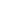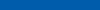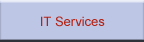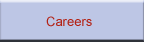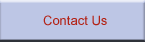Mathematical ModellingWe need mathematical models to understand the physical properties of a system and to be able to predict its behaviour. We are currently investigating the growth of an oxide layer, by mathematical models of a set of second order nonlinear parabolic partial differential equations (PDE). The solutions to these equations help us understand the behaviour of the oxide layer and the inititation of cracks. These cracks critcally affects the lifetime of TBCs (Thermal barrier coatings) and the reliability of the engines. We have extensive research experience in the area of mathematical modelling: Numerical Analysis and Simulation Solution of Partial Differential Equations TECSIS CORPORATION has collaborations with various Canadian Universities.